# High School Math : Understanding Vector Coordinates

## Example Questions

### Example Question #1 : Vector

Find the vector where its initial point is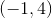and its terminal point is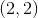.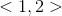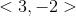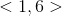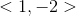Explanation:

We need to subtract the-coordinate and the-coordinates to solve for a vector when given its initial and terminal coordinates:

Initial pt:Terminal pt:Vector: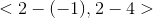Vector:### Example Question #6 : Calculus Ii — Integrals

Find the vector where its initial point is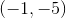and its terminal point is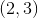.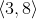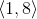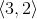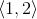Explanation:

We need to subtract the-coordinate and the-coordinate to solve for a vector when given its initial and terminal coordinates:

Initial pt:Terminal pt:Vector: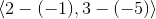Vector: